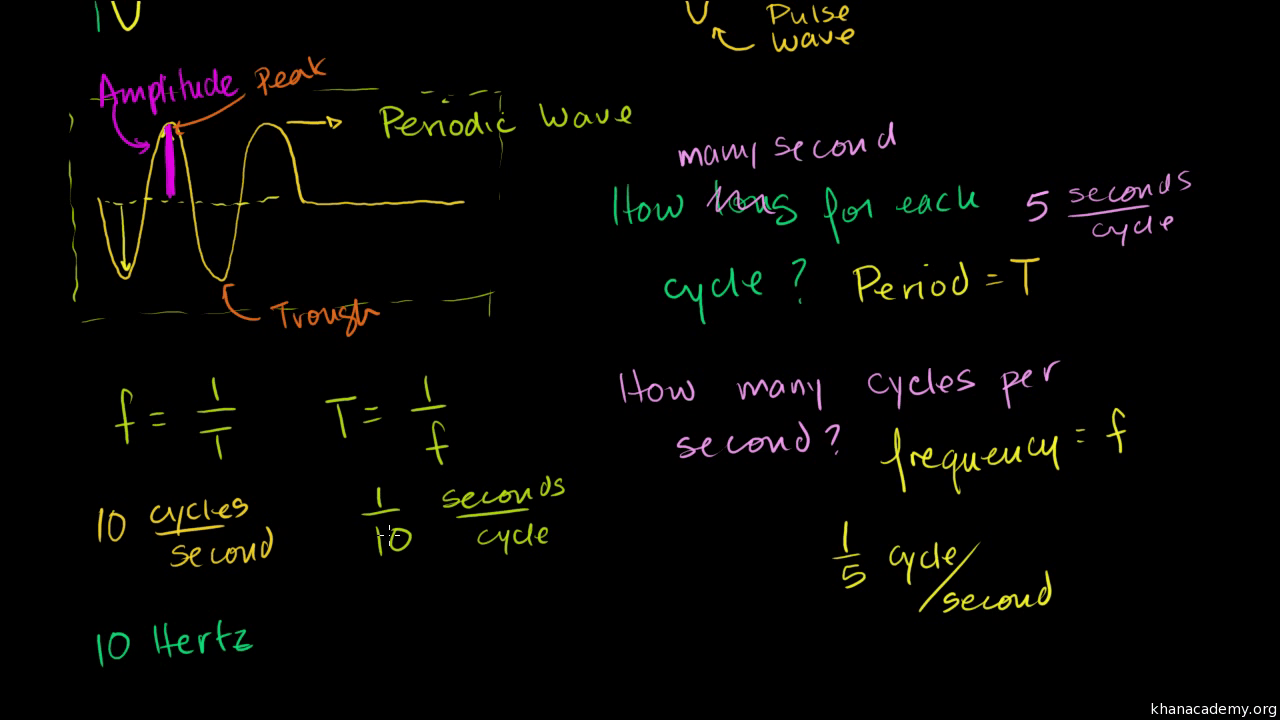Before understanding the wave motion, it is important to understand what is a wave. Wave is defined as the disturbance which transfers energy through matter or space. It consists of oscillations or vibrations.

## What are the types of waves?

There are two fundamental types of waves:

• Longitudinal waves: These waves consist of compressions and rarefactions and the particles are displaced parallel to the direction in which the wave travels. The waves observed in a slinky and sound wave in the air are examples of longitudinal waves.
• Transverse waves: These waves particles are displaced perpendicular to the direction in which the wave travels. A wave on a string and a ripple on a pond are examples of transverse waves.

## What is wave motion?

Wave motion is defined as the propagation of disturbances or vibrations from the equilibrium with a constant velocity. The understanding of wave motion began with the study of sound and vacuums.

## What are the factors of wave motion?

Irrespective of the types of waves, the factors of transverse and longitudinal waves are the same. Following are the list of factors of wave motions:

• Frequency: Frequency(f) of a wave is defined as the number of complete cycles taken by the waves in a specific period of time. As the frequency increases, the number of compressions and expansions in the waves increases. It is measured in Hertz and mathematically it is given as f=1/T
• Time period: The time period (T) of a wave is the time required to produce one complete cycle.
• Amplitude: The amplitude (A) of a wave is the maximum displacement of the vibrating particles. It is measured in meter (m).
• Wavelength: Wavelength (λ) of a wave is the distance between two points in a wave that is close to each other and in the same phase. It is denoted by λ.

## What are the characteristics of wave motion?

Following are the list of characteristics of wave motion;

• There is no transfer of matter through the medium, only the energy is transferred.
• The velocity at which the wave propagates is the same throughout the medium and is dependent on the nature of the medium.
• The medium must possess elasticity and inertia for the propagation of the waves.
• The vibration of the particles takes place at their equilibrium position.
• The particles possess kinetic energy as well as potential energy at their mean position and at extreme positions respectively.

Interested to learn more about what causes wave motion and other Physics related concepts like Simple Harmonic Motion, unit of magnetic flux, types of optical fibresetc, visit BYJU’S.